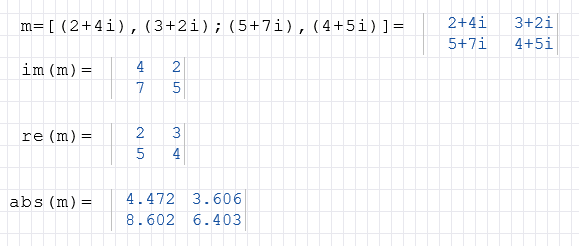Complex numbers

RedCrab Calculator and Complex numbers

Complex numbers basics

Available in RedCrab PLUS

• A complex number is expressed in the form 2+2i. The i after the second number marks the complex number imaginary part.

• The usual rules for real numbers can be applied to complex numbers.

• All trigonometric and logarithmic functions and the functions Abs, Power, Sqrt and Sqr work with complex numbers
• Complex numbers in lists or tables must be written in brackets.

The following example shows the definition of a 2x2 matrix and its use as an argument in different functions.Functions of complex numbers:

Re gets the real component of a complex number
Im gets the imaginary component of a complex number
Arg gets the angle of a complex number
Imag change a real number to a imaginary number
Complex converted a complex number from two real numbers.
Magnitude gets the magnitude (or absolute value) of a complex number
FromPolar creates a complex number from a point's polar coordinates
Conjugate computes the conjugate of a complex number